### 4.2. 指针的引入

Just as we can traverse a vector either by using a subscript or an iterator, we can also traverse an array by using either a subscript or a pointer. A pointer is a compound type; a pointer points to an object of some other type. Pointers are iterators for arrays: A pointer can point to an element in an array. The dereference and increment operators, when applied to a pointer that points to an array element, have similar behavior as when applied to an iterator. When we dereference a pointer, we obtain the object to which the pointer points. When we increment a pointer, we advance the pointer to denote the next element in the array. Before we write programs using pointers, we need to know a bit more about them.

vector 的遍历可使用下标或迭代器实现，同理，也可用下标或指针来遍历数组。指针是指向某种类型对象的复合数据类型，是用于数组的迭代器：指向数组中的一个元素。在指向数组元素的指针上使用解引用操作符 *（dereference operator）和自增操作符 ++（increment operator），与在迭代器上的用法类似。对指针进行解引用操作，可获得该指针所指对象的值。而当指针做自增操作时，则移动指针使其指向数组中的下一个元素。在使用指针编写程序之前，我们需进一步了解一下指针。

#### 4.2.1. 什么是指针

For newcomers, pointers are often hard to understand. Debugging problems due to pointer errors bedevil even experienced programmers. However, pointers are an important part of most C programs and to a much lesser extent remain important in many C++ programs.

Conceptually, pointers are simple: A pointer points at an object. Like an iterator, a pointer offers indirect access to the object to which it points. However, pointers are a much more general construct. Unlike iterators, pointers can be used to point at single objects. Iterators are used only to access elements in a container.

Specifically, a pointer holds the address of another object:

```          string s("hello world");
string *sp = &s; // sp holds the address of s
```

The second statement defines sp as a pointer to string and initializes sp to point to the string object named s. The * in *sp indicates that sp is a pointer. The & operator in &s is the address-of operator. It returns a value that when dereferenced yields the original object. The address-of operator may be applied only to an lvalue (Section 2.3.1, p. 45). Because a variable is an lvalue, we may take its address. Similarly, the subscript and dereference operators, when applied to a vector, string, or built-in array, yield lvalues. Because these operators yield lvalues, we may apply the address-of to the result of the subscript or dereference operator. Doing so gives us the address of a particular element.

## 建议：尽量避免使用指针和数组

Pointers and arrays are surprisingly error-prone. Part of the problem is conceptual: Pointers are used for low-level manipulations and it is easy to make bookkeeping mistakes. Other problems arise because of the syntax, particularly the declaration syntax used with pointers.

Many useful programs can be written without needing to use arrays or pointers. Instead, modern C++ programs should use vectors and iterators to replace general arrays and strings to replace C-style array-based character strings.

#### 4.2.2. 指针的定义和初始化

Every pointer has an associated type. The type of a pointer determines the type of the objects to which the pointer may point. A pointer to int, for example, may only point to an object of type int.

##### 指针变量的定义

We use the * symbol in a declaration to indicate that an identifier is a pointer:

C++ 语言使用 * 符号把一个标识符声明为指针：

```          vector<int>   *pvec;      // pvec can point to a vector<int>
int           *ip1, *ip2; // ip1 and ip2 can point to an int
string        *pstring;   // pstring can point to a string
double        *dp;        // dp can point to a double
```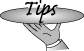When attempting to understand pointer declarations, read them from right to left. 理解指针声明语句时，请从右向左阅读。

Reading the definition of pstring from right to left, we see that

```          string *pstring;
```

defines pstring as a pointer that can point to string objects. Similarly,

```          int *ip1, *ip2; // ip1 and ip2 can point to an int
```

defines ip2 as a pointer and ip1 as a pointer. Both pointers point to ints.

ip1ip2 都定义为指向 int 型对象的指针。

The * can come anywhere in a list of objects of a given type:

```          double dp, *dp2; // dp2 is a ponter, dp is an object: both type double
```

defines dp2 as a pointer and dp as an object, both of type double.

##### 另一种声明指针的风格

The * symbol may be separated from its identifier by a space. It is legal to write:

```          string* ps; // legal but can be misleading
```

which says that ps is a pointer to string.

We say that this definition can be misleading because it encourages the belief that string* is the type and any variable defined in the same definition is a pointer to string. However,

```          string* ps1, ps2; // ps1 is a pointer to string,  ps2 is a string
```

defines ps1 as a pointer, but ps2 is a plain string. If we want to define two pointers in a single definition, we must repeat the * on each identifier:

```          string* ps1, *ps2; // both ps1 and ps2 are pointers to string
```
##### 连续声明多个指针易导致混淆

There are two common styles for declaring multiple pointers of the same type. One style requires that a declaration introduce only a single name. In this style, the * is placed with the type to emphasize that the declaration is declaring a pointer:

```          string* ps1;
string* ps2;
```

The other style permits multiple declarations in a single statement but places the * adjacent to the identifier. This style emphasizes that the object is a pointer:

```          string *ps1, *ps2;
```As with all questions of style, there is no single right way to declare pointers. The important thing is to choose a style and stick with it. 关于指针的声明，不能说哪种声明风格是唯一正确的方式，重要的是选择一种风格并持续使用。

In this book we use the second style and place the * with the pointer variable name.

##### 指针可能的取值

A valid pointer has one of three states: It can hold the address of a specific object, it can point one past the end of an object, or it can be zero. A zero-valued pointer points to no object. An uninitialized pointer is invalid until it is assigned a value. The following definitions and assignments are all legal:

```          int ival = 1024;
int *pi = 0;       // pi initialized to address no object
int *pi2 = & ival; // pi2 initialized to address of ival
int *pi3;          // ok, but dangerous, pi3 is uninitialized
pi = pi2;          // pi and pi2 address the same object, e.g. ival
pi2 = 0;           // pi2 now addresses no object
```
##### 避免使用未初始化的指针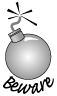Uninitialized pointers are a common source of run-time errors. 很多运行时错误都源于使用了未初始化的指针。

As with any other uninitialized variable, what happens when we use an uninitialized pointer is undefined. Using an uninitialized pointer almost always results in a run-time crash. However, the fact that the crash results from using an uninitialized pointer can be quite hard to track down.

Under most compilers, if we use an uninitialized pointer the effect will be to use whatever bits are in the memory in which the pointer resides as if it were an address. Using an uninitialized pointer uses this supposed address to manipulate the underlying data at that supposed location. Doing so usually leads to a crash as soon as we attempt to dereference the uninitialized pointer.

It is not possible to detect whether a pointer is uninitialized. There is no way to distinguish a valid address from an address formed from the bits that are in the memory in which the pointer was allocated. Our recommendation to initialize all variables is particularly important for pointers.

C++ 语言无法检测指针是否未被初始化，也无法区分有效地址和由指针分配到的存储空间中存放的二进制位形成的地址。建议程序员在使用之前初始化所有的变量，尤其是指针。If possible, do not define a pointer until the object to which it should point has been defined. That way, there is no need to define an uninitialized pointer. 如果可能的话，除非所指向的对象已经存在，否则不要先定义指针，这样可避免定义一个未初始化的指针。

If you must define a pointer separately from pointing it at an object, then initialize the pointer to zero. The reason is that a zero-valued pointer can be tested and the program can detect that the pointer does not point to an object.

##### 指针初始化和赋值操作的约束

There are only four kinds of values that may be used to initialize or assign to a pointer:

1. A constant expression (Section 2.7, p. 62) with value 0 (e.g., a const integral object whose value is zero at compile time or a literal constant 0)

0 值常量表达式（第 2.7 节），例如，在编译时可获得 0 值的整型 const 对象或字面值常量 0。

2. An address of an object of an appropriate type

类型匹配的对象的地址。

3. The address one past the end of another object

另一对象末的下一地址。

4. Another valid pointer of the same type

同类型的另一个有效指针。

It is illegal to assign an int to a pointer, even if the value of the int happens to be 0. It is okay to assign the literal 0 or a const whose value is known to be 0 at compile time:

int 型变量赋给指针是非法的，尽管此 int 型变量的值可能为 0。但允许把数值 0 或在编译时可获得 0 值的 const 量赋给指针：

```          int ival;
int zero = 0;
const int c_ival = 0;
int *pi = ival; // error: pi initialized from int value of ival
pi = zero;      // error: pi assigned int value of zero
pi = c_ival;    // ok: c_ival is a const with compile-time value of 0
pi = 0;         // ok: directly initialize to literal constant 0
```

In addition to using a literal 0 or a const with a compile-time value of 0, we can also use a facility that C++ inherits from C. The cstdlib header defines a preprocessor variable (Section 2.9.2, p. 69) named NULL, which is defined as 0. When we use a preprocessor variable in our code, it is automatically replaced by its value. Hence, initializing a pointer to NULL is equivalent to initializing it to 0:

```          // cstdlib #defines NULL to 0
int *pi = NULL; // ok: equivalent to int *pi = 0;
```

As with any preprocessor variable (Section 2.9.2, p. 71) we should not use the name NULL for our own variables.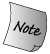Preprocessor variables are not defined in the std namespace and hence the name is NULL, not std::NULL. 预处理器变量不是在 std 命名空间中定义的，因此其名字应为 NULL，而非 std::NULL。

With two exceptions, which we cover in Sections 4.2.5 and 15.3, we may only initialize or assign a pointer from an address or another pointer that has the same type as the target pointer:

```          double dval;
double *pd = &dval;   // ok: initializer is address of a double
double *pd2 = pd;     // ok: initializer is a pointer to double

int *pi = pd;   // error: types of pi and pd differ
pi = &dval;     // error: attempt to assign address of a double to int *
```

The reason the types must match is that the type of the pointer is used to determine the type of the object that it addresses. Pointers are used to indirectly access an object. The operations that the pointer can perform are based on the type of the pointer: A pointer to int treats the underlying object as if it were an int. If that pointer actually addressed an object of some other type, such as double, then any operations performed by the pointer would be in error.

##### void* 指针

The type void* is a special pointer type that can hold an address of any object:

C++ 提供了一种特殊的指针类型 void*，它可以保存任何类型对象的地址：

```          double obj = 3.14;
double *pd = &obj;
// ok: void* can hold the address value of any data pointer type
void *pv = &obj;       // obj can be an object of any type
pv = pd;               // pd can be a pointer to any type
```

A void* indicates that the associated value is an address but that the type of the object at that address is unknown.

void* 表明该指针与一地址值相关，但不清楚存储在此地址上的对象的类型。

There are only a limited number of actions we can perform on a void* pointer: We can compare it to another pointer, we can pass or return it from a function, and we can assign it to another void* pointer. We cannot use the pointer to operate on the object it addresses. We'll see in Section 5.12.4 (p. 183) how we can retrieve the address stored in a void* pointer.

void* 指针只支持几种有限的操作：与另一个指针进行比较；向函数传递 void* 指针或从函数返回 void* 指针；给另一个 void* 指针赋值。不允许使用 void* 指针操纵它所指向的对象。我们将在第 5.12.4 节讨论如何重新获取存储在 void* 指针中的地址。

## Exercises Section 4.2.2

 Exercise 4.10: Explain the rationale for preferring the first form of pointer declaration: 下面提供了两种指针声明的形式，解释宁愿使用第一种形式的原因： ``` int *ip; // good practice int* ip; // legal but misleading ``` Exercise 4.11: Explain each of the following definitions. Indicate whether any are illegal and if so why. 解释下列声明语句，并指出哪些是非法的，为什么？ ``` (a) int* ip; (b) string s, *sp = 0; (c) int i; double* dp = &i; (d) int* ip, ip2; (e) const int i = 0, *p = i; (f) string *p = NULL; ``` Exercise 4.12: Given a pointer, p, can you determine whether p points to a valid object? If so, how? If not, why not? 已知一指针 p，你可以确定该指针是否指向一个有效的对象吗？如果可以，如何确定？如果不可以，请说明原因。 Exercise 4.13: Why is the first pointer initialization legal and the second illegal? 下列代码中，为什么第一个指针的初始化是合法的，而第二个则不合法？ ``` int i = 42; void *p = &i; long *lp = &i; ```

#### 4.2.3. 指针操作

Pointers allow indirect manipulation of the object to which the pointer points. We can access the object by dereferencing the pointer. Dereferencing a pointer is similar to dereferencing an iterator (Section 3.4, p. 98). The * operator (the dereference operator) returns the object to which the pointer points:

```          string s("hello world");
string *sp = &s; // sp holds the address of s
cout  <<*sp;     // prints hello world
```

When we dereference sp, we fetch the value of s. We hand that value to the output operator. The last statement, therefore, prints the contents of sthat is, hello world.

sp 进行解引用将获得 s 的值，然后用输出操作符输出该值，于是最后一条语句输出了 s 的内容 hello world

##### 生成左值的解引用操作

The dereference operator returns the lvalue of the underlying object, so we can use it to change the value of the object to which the pointer points:

```          *sp = "goodbye"; // contents of s now changed
```

Because we assign to *sp, this statement leaves sp pointing to s and changes the value of s.

We can also assign a new value to sp itself. Assigning to sp causes sp to point to a different object:

```          string s2 = "some value";
sp = &s2;  // sp now points to s2
```

We change the value of a pointer by assigning to it directlywithout dereferencing the pointer.

## 关键概念：给指针赋值或通过指针进行赋值

When first using pointers, the difference in whether an assignment is to the pointer or through the pointer to the value pointed to can be confusing. The important thing to keep in mind is that if the left-hand operand is dereferenced, then the value pointed to is changed. If there is no dereference, then the pointer itself is being changed. A picture can sometimes help: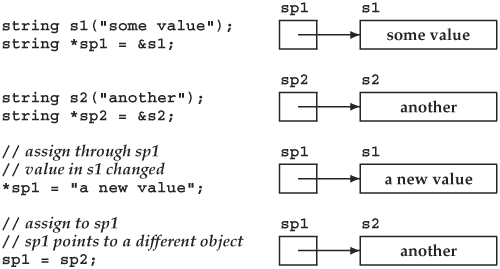##### 指针和引用的比较

While both references and pointers are used to indirectly access another value, there are two important differences between references and pointers. The first is that a reference always refers to an object: It is an error to define a reference without initializing it. The behavior of assignment is the second important difference: Assigning to a reference changes the object to which the reference is bound; it does not rebind the reference to another object. Once initialized, a reference always refers to the same underlying object.

Consider these two program fragments. In the first, we assign one pointer to another:

```          int ival = 1024, ival2 = 2048;
int *pi = &ival, *pi2 = &ival2;
pi = pi2;    // pi now points to ival2
```

After the assignment, ival, the object addressed by pi remains unchanged. The assignment changes the value of pi, making it point to a different object. Now consider a similar program that assigns two references:

```          int &ri = ival, &ri2 = ival2;
ri = ri2;    // assigns ival2 to ival
```

This assignment changes ival, the value referenced by ri, and not the reference itself. After the assignment, the two references still refer to their original objects, and the value of those objects is now the same as well.

##### 指向指针的指针

Pointers are themselves objects in memory. They, therefore, have addresses that we can store in a pointer:

```          int ival = 1024;
int *pi = &ival; // pi points to an int
int **ppi = &pi; // ppi points to a pointer to int
```

which yields a pointer to a pointer. We designate a pointer to a pointer by using **. We might represent these objects as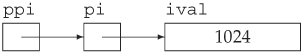As usual, dereferencing ppi yields the object to which ppi points. In this case, that object is a pointer to an int:

ppi 进行解引用照常获得 ppi 所指的对象，在本例中，所获得的对象是指向 int 型变量的指针 pi

```          int *pi2 = *ppi; // ppi points to a pointer
```

To actually access ival, we need to dereference ppi twice:

```          cout << "The value of ival\n"
<< "direct value: " << ival << "\n"
<< "indirect value: " << *pi << "\n"
<< "doubly indirect value: " << **ppi
<< endl;
```

This program prints the value of ival three different ways. First, by direct reference to the variable. Then, through the pointer to int in pi, and finally, by dereferencing ppi twice to get to the underlying value in ival.

## Exercises Section 4.2.3

 Exercise 4.14: Write code to change the value of a pointer. Write code to change the value to which the pointer points. 编写代码修改指针的值；然后再编写代码修改指针所指对象的值。 Exercise 4.15: Explain the key differences between pointers and references. 解释指针和引用的主要区别。 Exercise 4.16: What does the following program do? 下列程序段实现什么功能？ ``` int i = 42, j = 1024; int *p1 = &i, *p2 = &j; *p2 = *p1 * *p2; *p1 *= *p1; ```

#### 4.2.4. 使用指针访问数组元素

Pointers and arrays are closely intertwined in C++. In particular, when we use the name of an array in an expression, that name is automatically converted into a pointer to the first element of the array:

C++ 语言中，指针和数组密切相关。特别是在表达式中使用数组名时，该名字会自动转换为指向数组第一个元素的指针：

```          int ia[] = {0,2,4,6,8};
int *ip = ia; // ip points to ia
```

If we want to point to another element in the array, we could do so by using the subscript operator to locate the element and then applying the address-of operator to find its location:

```          ip = &ia;    // ip points to last element in ia
```
##### 指针的算术操作

Rather than taking the address of the value returned by subscripting, we could use pointer arithmetic. Pointer arithmetic works the same way (and has the same constraints) as iterator arithmetic (Section 3.4.1, p. 100). Using pointer arithmetic, we can compute a pointer to an element by adding (or subtracting) an integral value to (or from) a pointer to another element in the array:

```          ip = ia;            // ok: ip points to ia
int *ip2 = ip + 4;  // ok: ip2 points to ia, the last element in ia
```

When we add 4 to the pointer ip, we are computing a new pointer. That new pointer points to the element four elements further on in the array from the one to which ip currently points.

More generally, when we add (or subtract) an integral value to a pointer, the effect is to compute a new pointer. The new pointer points to the element as many elements as that integral value ahead of (or behind) the original pointer.Pointer arithmetic is legal only if the original pointer and the newly calculated pointer address elements of the same array or an element one past the end of that array. If we have a pointer to an object, we can also compute a pointer that points just after that object by adding one to the pointer. 指针的算术操作只有在原指针和计算出来的新指针都指向同一个数组的元素，或指向该数组存储空间的下一单元时才是合法的。如果指针指向一对象，我们还可以在指针上加1从而获取指向相邻的下一个对象的指针。

Given that ia has 4 elements, adding 10 to ia would be an error:

```          // error: ia has only 4 elements, ia + 10 is an invalid address
int *ip3 = ia + 10;
```

We can also subtract two pointers as long as they point into the same array or to an element one past the end of the array:

```          ptrdiff_t n = ip2 - ip; // ok: distance between the pointers
```

The result is four, the distance between the two pointers, measured in objects. The result of subtracting two pointers is a library type named ptrdiff_t. Like size_t, the ptrdiff_t type is a machine-specific type and is defined in the cstddef header. The size_t type is an unsigned type, whereas ptrdiff_t is a signed integral type.

The difference in type reflects how these two types are used: size_t is used to hold the size of an array, which must be a positive value. The ptrdiff_t type is guaranteed to be large enough to hold the difference between any two pointers into the same array, which might be a negative value. For example, had we subtracted ip2 from ip, the result would be -4.

It is always possible to add or subtract zero to a pointer, which leaves the pointer unchanged. More interestingly, given a pointer that has a value of zero, it is also legal to add zero to that pointer. The result is another zero-valued pointer. We can also subtract two pointers that have a value of zero. The result of subtracting two zero-valued pointers is zero.

##### 解引用和指针算术操作之间的相互作用

The result of adding an integral value to a pointer is itself a pointer. We can dereference the resulting pointer directly without first assigning it to another pointer:

```          int last = *(ia + 4); // ok: initializes last to 8, the value of ia
```

This expression calculates the address four elements past ia and dereferences that pointer. It is equivalent to writing ia.The parentheses around the addition are essential. Writing 加法操作两边用圆括号括起来是必要的。如果写为：

```          last = *ia + 4;     // ok: last = 4, equivalent to ia+4
```

means dereference ia and add four to the dereferenced value.

The parentheses are required due to the precedence of the addition and dereference operators. We'll learn more about precedence in Section 5.10.1 (p. 168). Simply put, precedence stipulates how operands are grouped in expressions with multiple operators. The dereference operator has a higher precedence than the addition operator.

The operands to operators with higher precedence are grouped more tightly than those of lower precedence. Without the parentheses, the dereference operator would use ia as its operand. The expression would be evaluated by dereferencing ia and adding four to the value of the element at the beginning of ia.

By parenthesizing the expression, we override the normal precedence rules and effectively treat (ia + 4) as a single operand. That operand is an address of an element four past the one to which ia points. That new address is dereferenced.

##### 下标和指针

We have already seen that when we use an array name in an expression, we are actually using a pointer to the first element in the array. This fact has a number of implications, which we shall point out as they arise.

One important implication is that when we subscript an array, we are really subscripting a pointer:

```          int ia[] = {0,2,4,6,8};
int i = ia; // ia points to the first element in ia
```

When we write ia, that is an expression that uses the name of an array. When we subscript an array, we are really subscripting a pointer to an element in that array. We can use the subscript operator on any pointer, as long as that pointer points to an element in an array:

ia 是一个使用数组名的表达式。在使用下标访问数组时，实际上是对指向数组元素的指针做下标操作。只要指针指向数组元素，就可以对它进行下标操作：

```          int *p = &ia;     // ok: p points to the element indexed by 2
int j = p;        // ok: p equivalent to *(p + 1),
//    p is the same element as ia
int k = p[-2];       // ok: p[-2] is the same element as ia
```
##### 计算数组的超出末端指针

When we use a vector, the end operation returns an iterator that refers just past the end of the vector. We often use this iterator as a sentinel to control loops that process the elements in the vector. Similarly, we can compute an off-the-end pointer value:

vector 类型提供的 end 操作将返回指向超出 vector 末端位置的一个迭代器。这个迭代器常用作哨兵，来控制处理 vector 中元素的循环。类似地，可以计算数组的超出末端指针的值：

```          const size_t arr_size = 5;
int arr[arr_size] = {1,2,3,4,5};
int *p = arr;           // ok: p points to arr
int *p2 = p + arr_size; // ok: p2 points one past the end of arr
//    use caution -- do not dereference!
```

In this case, we set p to point to the first element in arr. We then calculate a pointer one past the end of arr by adding the size of arr to the pointer value in p. When we add 5 to p, the effect is to calculate the address of that is five ints away from pin other words, p + 5 points just past the end of arr.It is legal to compute an address one past the end of an array or object. It is not legal to dereference a pointer that holds such an address. Nor is it legal to compute an address more than one past the end of an array or an address before the beginning of an array. C++ 允许计算数组或对象的超出末端的地址，但不允许对此地址进行解引用操作。而计算数组超出末端位置之后或数组首地址之前的地址都是不合法的。

The address we calculated and stored in p2 acts much like the iterator returned from the end operation on vectors. The iterator we obtain from end denotes "one past the end" of the vector. We may not dereference that iterator, but we may compare it to another iterator value to see whether we have processed all the elements in the vector. Similarly, the value we calculated for p2 can be used only to compare to another pointer value or as an operand in a pointer arithmetic expression. If we attempt to dereference p2, the most likely result is that it would yield some garbage value. Most compilers, would treat the result of dereferencing p2 as an int, using whatever bits happened to be in memory at the location just after the last element in arr.

##### 输出数组元素

Now we are ready to write a program that uses pointers:

```          const size_t arr_sz = 5;
int int_arr[arr_sz] = { 0, 1, 2, 3, 4 };
// pbegin points to first element, pend points just after the last
for (int *pbegin = int_arr, *pend = int_arr + arr_sz;
pbegin != pend; ++pbegin)
cout << *pbegin << ' '; // print the current element
```

This program uses a feature of the for loop that we have not yet used: We may define multiple variables inside the init-statement (Section 1.4.2, p. 14) of a for as long as the variables are defined using the same type. In this case, we're defining two int pointers named pbegin and pend.

We use these pointers to traverse the array. Like other built-in types, arrays have no member functions. Hence, there are no begin and end operations on arrays. Instead, we must position pointers to denote the first and one past the last elements ourselves. We do so in the initialization of our two pointers. We initialize pbegin to address the first element of int_arr and pend to one past the last element in the array:

C++ 允许使用指针遍历数组。和其他内置类型一样，数组也没有成员函数。因此，数组不提供 beginend 操作，程序员只能自己给指针定位，使之分别标志数组的起始位置和超出末端位置。可在初始化中实现这两个指针的定位：初始化指针 pbegin 指向 int_arr 数组的第一个元素，而指针 pend 则指向该数组的超出末端的位置：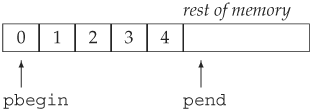The pointer pend serves as a sentinel, allowing the for loop to know when to stop. Each iteration of the for loop increments pbegin to address the next element. On the first trip through the loop, pbegin denotes the first element, on the second iteration, the second element, and so on. After processing the last element in the array, pbegin will be incremented once more and will then equal pend. At that point we know that we have iterated across the entire array.

##### 指针是数组的迭代器

Astute readers will note that this program is remarkably similar to the program on page 99, which traversed and printed the contents of a vector of strings. The loop in that program

```          // equivalent loop using iterators to reset all the elements in ivec to 0
for (vector<int>::iterator iter = ivec.begin();
iter != ivec.end(); ++iter)
*iter = 0; // set element to which iter refers to 0
```

used iterators in much the same way that pointers are used in the program to print the contents of the array. This similarity is not a coincidence. In fact, the built-in array type has many of the properties of a library container, and pointers, when we use them in conjunction with arrays, are themselves iterators. We'll have much more to say about containers and iterators in Part II.

## Exercises Section 4.2.4

 Exercise 4.17: Given that p1 and p2 point to elements in the same array, what does the following statement do? 已知 p1 和 p2 指向同一个数组中的元素，下面语句实现什么功能？ ``` p1 += p2 - p1; ``` Are there any values of p1 or p2 that could make this code illegal? 当 p1 和 p2 具有什么值时这个语句是非法的？ Exercise 4.18: Write a program that uses pointers to set the elements in an array of ints to zero. 编写程序，使用指针把一个 int 型数组的所有元素设置为0。

#### 4.2.5. 指针和 const 限定符

There are two kinds of interactions between pointers and the const qualifier discussed in Section 2.4 (p. 56): We can have pointers to const objects and pointers that are themselves const. This section discusses both kinds of pointers.

##### 指向 const 对象的指针

The pointers we've seen so far can be used to change the value of the objects to which they point. But if we have a pointer to a const object, we do not want to allow that pointer to change the underlying, const value. The language enforces this property by requiring that pointers to const objects must take the constness of their target into account:

```          const double *cptr;  // cptr may point to a double that is const
```

Here cptr is a pointer to an object of type const double. The const qualifies the type of the object to which cptr points, not cptr itself. That is, cptr itself is not const. We need not initialize it and can assign a new value to it if we so desire. What we cannot do is use cptr to change the value to which it points:

```          *cptr = 42;   // error: *cptr might be const
```

It is also a compile-time error to assign the address of a const object to a plain, nonconst pointer:

```          const double pi = 3.14;
double *ptr = &pi;        // error: ptr is a plain pointer
const double *cptr = &pi; // ok: cptr is a pointer to const
```

We cannot use a void* pointer (Section 4.2.2, p. 119) to hold the address of a const object. Instead, we must use the type const void* to hold the address of a const object:

```          const int universe = 42;
const void *cpv = &universe; // ok: cpv is const
void *pv = &universe;        // error: universe is const
```

A pointer to a const object can be assigned the address of a nonconst object, such as

```          double dval = 3.14; // dval is a double; its value can be changed
cptr = &dval;       // ok: but can't change dval through cptr
```

Although dval is not a const, any attempt to modify its value through cptr results in a compile-time error. When we declared cptr, we said that it would not change the value to which it points. The fact that it happens to point to a nonconst object is irrelevant.We cannot use a pointer to const to change the underlying object. However, if the pointer addresses a nonconst object, it is possible that some other action will change the object to which the pointer points. 不能使用指向 const 对象的指针修改基础对象，然而如果该指针指向的是一个非 const 对象，可用其他方法修改其所指的对象。

The fact that values to which a const pointer points can be changed is subtle and can be confusing. Consider:

```          dval = 3.14159;       // dval is not const
*cptr = 3.14159;      // error: cptr is a pointer to const
double *ptr = &dval;  // ok: ptr points at non-const double
*ptr = 2.72;          // ok: ptr is plain pointer
cout << *cptr;        // ok: prints 2.72
```

In this case, cptr is defined as a pointer to const but it actually points at a nonconst object. Even though the object to which it points is nonconst, we cannot use cptr to change the object's value. Essentially, there is no way for cptr to know whether the object it points to is const, and so it treats all objects to which it might point as const.

When a pointer to const does point to a nonconst, it is possible that the value of the object might change: After all, that value is not const. We could either assign to it directly or, as here, indirectly through another, plain nonconst pointer. It is important to remember that there is no guarantee that an object pointed to by a pointer to const won't change.It may be helpful to think of pointers to const as "pointers that think they point to const." 如果把指向 const 的指针理解为“自以为指向 const 的指针”，这可能会对理解有所帮助。

In real-world programs, pointers to const occur most often as formal parameters of functions. Defining a parameter as a pointer to const serves as a contract guaranteeing that the actual object being passed into the function will not be modified through that parameter.

##### const 指针

In addition to pointers to const, we can also have const pointersthat is, pointers whose own value we may not change:

```          int errNumb = 0;
int *const curErr = &errNumb; // curErr is a constant pointer
```

Reading this definition from right to left, we see that "curErr is a constant pointer to an object of type int." As with any const, we may not change the value of the pointerthat is, we may not make it point to any other object. Any attempt to assign to a constant pointereven assigning the same value back to curErris flagged as an error during compilation:

```          curErr = curErr; // error: curErr is const
```

As with any const, we must initialize a const pointer when we create it.

The fact that a pointer is itself const says nothing about whether we can use the pointer to change the value to which it points. Whether we can change the value pointed to depends entirely on the type to which the pointer points. For example, curErr addresses a plain, nonconst int. We can use curErr to change the value of errNumb:

```          if (*curErr) {
errorHandler();
*curErr = 0; // ok: reset value of the object to which curErr is bound
}
```
##### 指向 const 对象的 const 指针

We can also define a constant pointer to a constant object as follows:

```          const double pi = 3.14159;
// pi_ptr is const and points to a const object
const double *const pi_ptr = &pi;
```

In this case, neither the value of the object addressed by pi_ptr nor the address itself can be changed. We can read its definition from right to left as "pi_ptr is a constant pointer to an object of type double defined as const."

##### 指针和 typedef

The use of pointers in typedefs (Section 2.6, p. 61) often leads to surprising results. Here is a question almost everyone answers incorrectly at least once. Given the following,

```          typedef string *pstring;
const pstring cstr;
```

what is the type of cstr? The simple answer is that it is a pointer to const pstring. The deeper question is: what underlying type does a pointer to const pstring represent? Many think that the actual type is

```          const string *cstr; // wrong interpretation of const pstring cstr
```

That is, that a const pstring would be a pointer to a constant string. But that is incorrect.

The mistake is in thinking of a typedef as a textual expansion. When we declare a const pstring, the const modifies the type of pstring, which is a pointer. Therefore, this definition declares cstr to be a const pointer to string. The definition is equivalent to

```          // cstr is a const pointer to string
string *const cstr; // equivalent to const pstring cstr
```

## 建议：理解复杂的 const 类型的声明

Part of the problem in reading const declarations arises because the const can go either before or after the type:

```          string const s1;   // s1 and s2 have same type,
const string s2;   // they're both strings that are const
```

When writing const definitions using typedefs, the fact that the const can precede the type can lead to confusion as to the actual type being defined:

typedefconst 类型定义时，const 限定符加在类型名前面容易引起对所定义的真正类型的误解：

```[View full width]
string s;
typedef string *pstring;
const pstring cstr1 = &s; // written this way the type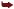is obscured
pstring const cstr2 = &s; // all three decreations arethe same type
string *const cstr3 = &s; // they're all const pointersto string
```

Putting the const after pstring and reading the declaration from right to left makes it clearer that cstr2 is a const pstring, which in turn is a const pointer to string.

const 放在类型 pstring 之后，然后从右向左阅读该声明语句就会非常清楚地知道 cstr2const pstring 类型，即指向 string 对象的 const 指针。

Unfortunately, most readers of C++ programs expect to see the const before the type. As a result, it is probably a good idea to put the const first, respecting common practice. But it can be helpful in understanding declarations to rewrite them to put the const after the type.

## Exercises Section 4.3

 Exercise 4.19: Explain the meaning of the following five definitions. Identify any illegal definitions. 解释下列 5 个定义的含义，指出其中哪些定义是非法的： ``` (a) int i; (b) const int ic; (c) const int *pic; (d) int *const cpi; (e) const int *const cpic; ``` Exercise 4.20: Which of the following initializations are legal? Explain why. 下列哪些初始化是合法的？为什么？ ``` (a) int i = -1; (b) const int ic = i; (c) const int *pic = ⁣ (d) int *const cpi = ⁣ (e) const int *const cpic = ⁣ ``` Exercise 4.21: Based on the definitions in the previous exercise, which of the following assignments are legal? Explain why. 根据上述定义，下列哪些赋值运算是合法的？为什么？ ``` (a) i = ic; (b) pic = ⁣ (c) cpi = pic; (d) pic = cpic; (e) cpic = ⁣ (f) ic = *cpic; ```